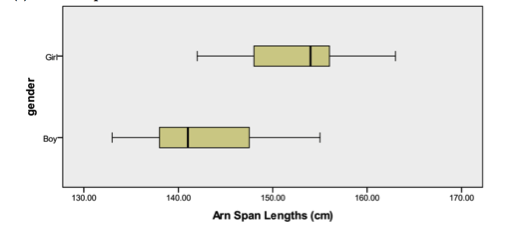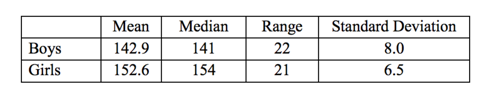# Descriptive Statistics

## Objective

Develop and answer statistical questions through data analysis of existing data using appropriate statistical measures and displays. (Part 3/3)

## Common Core Standards

### Core Standards

?

• HSS-ID.A.1 — Represent data with plots on the real number line (dot plots, histograms, and box plots).

• HSS-ID.A.2 — Use statistics appropriate to the shape of the data distribution to compare center (median, mean) and spread (interquartile range, standard deviation) of two or more different data sets.

• HSS-ID.A.3 — Interpret differences in shape, center, and spread in the context of the data sets, accounting for possible effects of extreme data points (outliers).

• HSS-ID.A.4 — Use the mean and standard deviation of a data set to fit it to a normal distribution and to estimate population percentages. Recognize that there are data sets for which such a procedure is not appropriate. Use calculators, spreadsheets, and tables to estimate areas under the normal curve.

?

• 7.SP.A.1

• 7.SP.A.2

• 7.SP.B.3

• 7.SP.B.4

## Criteria for Success

?

1. Develop a succinct yet informative written conclusion that clearly answers your statistical questions.
2. Identify calculations and other statistical evidence that support your solution.
3. Use statistical definitions to support your solution.
4. Use graphical analysis to support your solution.

## Tips for Teachers

?

• This is the third of three lessons that is project based. There is some guidance in the anchor problems that help to guide the project, but the data sets and questions can be swapped out.
• Additional data sets and options are included in the problem set guidance (same as in lesson 10).
• The target task for this lesson is a reflection of the work done in class and is not meant to be an assessment of how well students have completed this part of the project.

## Anchor Problems

?

Below is a sample graph and statistical measures.#### References

STatistics Education Web (STEW) Grades 6-8, Armspans

## Problem Set

?

The following resources include problems and activities aligned to the objective of the lesson that can be used to create your own problem set.